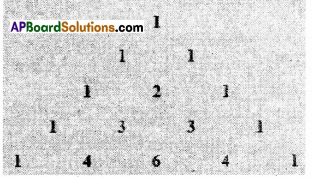# AP Board 9th Class Maths Solutions Chapter 15 Proofs in Mathematics Ex 15.3

AP State Syllabus AP Board 9th Class Maths Solutions Chapter 15 Proofs in Mathematics Ex 15.3 Textbook Questions and Answers.

## AP State Syllabus 9th Class Maths Solutions 15th Lesson Proofs in Mathematics Exercise 15.3Question 1.
Take any three consecutive odd numbers and find their product, for example 1 × 3 × 5 = 15;
3 × 5 × 7 = 105: 5 × 7 × 9 = ……………
ii) Take any three consecutive even numbers and add them, say,
2 + 4 + 6 = 12; 4 + 6 + 8 = 18:
6 + 8 + 10 = 24; 8 + 10 + 12 = 30 ….
so on. Is there any pattern you can guess in these sums ? What can von conjecture about them ?
Solution:
i) 1 × 3 × 5 = 15
3 × 5× 7 = 105
5 × 7 × 9 = 315
7 × 9 × 11 = 693

1. The product of any three consecutive odd numbers is odd.
2. The product of any three consecutive odd numbers is divisible by ’3′.
3. 2 + 4 + 6 = 12; 4 + 6 + 8= 18;
6 + 8 + 10 = 24; 8 + 10 + 12 = 30
4. The sum of any three consecutive even numbers is even.
5. The sum of any three consecutive even numbers is divisible by 6
6. The sum of any three consecutive even numbers is a multiple of 6.Question 2.
Go back to Pascal’s triangle.
Line-1: 1=11°
Line-2: 11 = 111
Line-3 : 121 = 112
Make a conjecture about line – 4 and line – 5.Does your conjecture hold ? Does your conjecture hold for line – 6 too ?
Solution:
Line-4 : 1331 = 113
Line-5 : 14641 = 114
Line – 6 : 115
∴ Line – n = 11n-1
Yes the conjecture holds good for line – 6 too.Question 3.
Look at the following pattern.
i) 28 = 22 × 71;
Total number of factors
(2+ 1)(1 + 1) = 3 × 2 = 6
28 is divisible by 6 factors i.e.,
1, 2, 4, 7, 14, 28.
ii) 30 = 21 × 31 × 51, Total number of .
factors (1 + 1) (1 + 1) (1 + 1) = 2 × 2 × 2 = 8
30 Is divisible by 8 factors i.e., 1, 2, 3, 5, 6, 10, 15 and 30
Find the pattern.
[Hint : Product of every prime base exponent +1)
Solution:
24 = 23 × 31
24 has (3+1) (1 + 1) = 4 × 2 = 8 factors
[1, 2, 3, 4, 6, 8, 12 and 24]
36 = 22 × 32
Number of factors = (2 + 1) (2 + 1)
3 × 3 = 9 [ 1, 2, 3. 4, 6, 9, 12, 18 and 36]
If N = ap. bq . cr…….. where N is a natural number.
a. b, c … are primes and p, q, r ……. are positive integers then, the number of factors of N =(p- 1)(q+ 1)(r + 1)Question 4.
Look at the following pattern :
12 = 1
112 = 121
1112 = 12321;
11112 = 1234321
111112 = 123454321
Make a conjecture about each of the following
1111112 =
11111112 =
Check if your conjecture is true.
Solution:
1111112 = 12345654321
11111112 = 1234567654321
(111………. n-times)2 = (123 … (n- 1) n (n – 1) (n – 2) 1)
The conjecture is true.Question 5.
List five axioms (postulates) used in text book.
Solution:

1. Things which are equal to the same things are equal to one another.
2. If equals are added to equals, the sums are equal.
3. If equals are subtracted from equals, the differences are equal.
4. When a pair of parallel lines are in-tersected by a transversal, the pairs of corresponding angles are equal.
5. There passes infinitely many lines through a given point.Question 6.
In a polynomial p(x) = x2 + x + 41 put different values of x and find p(x). Can you conclude after putting different values of x that p(x) is prime for all. Is ‘x’ an element of N ? Put x = 41 in p(x). Now what do you find ?
Solution:
p(x) = x2 + x + 41
p(0) = 02 + 0 + 41 = 41 – is a prime
p(1) = 12 + 1 + 41 = 43 – is a prime
p(2) = 22 + 2 + 41 = 47 – is a prime
p(3) = 32 + 3 + 41 = 53 – is a prime
p(41) = 412 + 41 + 41
= 41(41 + 1 + 1)
= 41 x 43 is not a prime.
∴ p(x) = x2+ x + 41 is not a prime for all x.
∴ The conjecture “p(x) = x2 + x + 41 is a prime” is false.